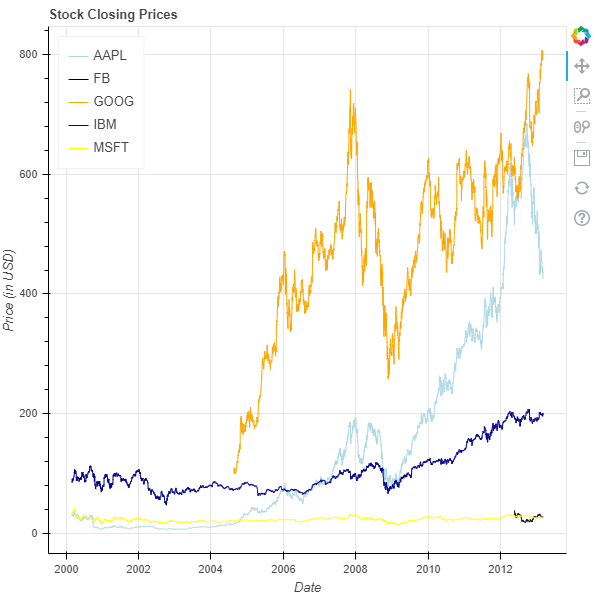# Python Bokeh – Visualizing Stock Data

Bokeh is a Python interactive data visualization. It renders its plots using HTML and JavaScript. It targets modern web browsers for presentation providing elegant, concise construction of novel graphics with high-performance interactivity.
Bokeh can be used to visualize stock market data. Visualization is be done using the `plotting` module. Here we will be using the sample stock datasets given to us by Bokeh.

To download the sample datasets run the following command on the command line :

`bokeh sampledata`

Alternatively, we can also execute the following Python code :

```import bokeh
```

## Analyzing the dataset :

In the sample data provided by Bokeh, there are datasets of the stocks of the following companies :

• AAPL which is Apple
• IBM which is International Business Machines
• MSFT which is Microsoft Corporation

All these datasets are available as CSV files. Below is a glimpse into the IBM.csv file :

```Date        Open    High    Low    Close    Volume        Adj Close
01-03-2000    102    105.5    100.06    100.25    10807800    84.48
02-03-2000    100.5    105.44    99.5    103.12    11192900    86.9
03-03-2000    107.25    110    106.06    108    10162800    91.01
06-03-2000    109.94    111    101    103.06    10747400    86.85
07-03-2000    106    107    101.69    103    10035100    86.8
```

The file contains the stock data between the years 2000 and 2013 with over 3000 entries.

## Visualizing the Stocks :

We will be plotting a line graph which will track the closing price of the stocks between the years 2000 and 2013 of all the 5 available companies.

1. Import the required modules :
• numpy
• figure, output_file and show from bokeh.plotting
• AAPL, FB, GOOG, IBM and MSFT from bokeh.sampledata.stocks
2. Instantiate a figure object with the title and axis types.
3. Give the names to x-axis and y-axis.
4. Plot line graphs for all the 5 companies.
5. Display the model.

 `# importing the modules ` `import` `numpy as np ` `from` `bokeh.plotting ``import` `figure, output_file, show ` `from` `bokeh.sampledata.stocks ``import` `AAPL, FB, GOOG, IBM, MSFT ` `  `  `# the file to save the model ` `output_file(``"gfg.html"``) ` `  `  `# instantiating the figure object ` `graph ``=` `figure(x_axis_type ``=` `"datetime"``, title ``=` `"Stock Closing Prices"``) ` `  `  `# name of the x-axis ` `graph.xaxis.axis_label ``=` `'Date'` `  `  `# name of the y-axis ` `graph.yaxis.axis_label ``=` `'Price (in USD)'` `  `  `# plotting the line graph for AAPL ` `x_axis_coordinates ``=` `np.array(AAPL[``'date'``], dtype ``=` `np.datetime64) ` `y_axis_coordinates ``=` `AAPL[``'adj_close'``] ` `color ``=` `"lightblue"` `legend_label ``=` `'AAPL'` `graph.line(x_axis_coordinates, ` `        ``y_axis_coordinates, ` `        ``color ``=` `color, ` `        ``legend_label ``=` `legend_label) ` `  `  `# plotting the line graph for FB ` `x_axis_coordinates ``=` `np.array(FB[``'date'``], dtype ``=` `np.datetime64) ` `y_axis_coordinates ``=` `FB[``'adj_close'``] ` `color ``=` `"black"` `legend_label ``=` `'FB'` `graph.line(x_axis_coordinates, ` `        ``y_axis_coordinates, ` `        ``color ``=` `color, ` `        ``legend_label ``=` `legend_label) ` `  `  `# plotting the line graph for GOOG ` `x_axis_coordinates ``=` `np.array(GOOG[``'date'``], dtype ``=` `np.datetime64) ` `y_axis_coordinates ``=` `GOOG[``'adj_close'``] ` `color ``=` `"orange"` `legend_label ``=` `'GOOG'` `graph.line(x_axis_coordinates, ` `        ``y_axis_coordinates, ` `        ``color ``=` `color, ` `        ``legend_label ``=` `legend_label) ` `  `  `# plotting the line graph for IBM ` `x_axis_coordinates ``=` `np.array(IBM[``'date'``], dtype ``=` `np.datetime64) ` `y_axis_coordinates ``=` `IBM[``'adj_close'``] ` `color ``=` `"darkblue"` `legend_label ``=` `'IBM'` `graph.line(x_axis_coordinates, ` `        ``y_axis_coordinates, ` `        ``color ``=` `color, ` `        ``legend_label ``=` `legend_label) ` `  `  `# plotting the line graph for MSFT ` `x_axis_coordinates ``=` `np.array(MSFT[``'date'``], dtype ``=` `np.datetime64) ` `y_axis_coordinates ``=` `MSFT[``'adj_close'``] ` `color ``=` `"yellow"` `legend_label ``=` `'MSFT'` `graph.line(x_axis_coordinates, ` `        ``y_axis_coordinates, ` `        ``color ``=` `color, ` `        ``legend_label ``=` `legend_label) ` `  `  `# relocating the legend table to  ` `# avoid abstruction of the graph ` `graph.legend.location ``=` `"top_left"` `  `  `# displaying the model ` `show(graph) `

Output :My Personal Notes arrow_drop_upCheck out this Author's contributed articles.

If you like GeeksforGeeks and would like to contribute, you can also write an article using contribute.geeksforgeeks.org or mail your article to contribute@geeksforgeeks.org. See your article appearing on the GeeksforGeeks main page and help other Geeks.

Please Improve this article if you find anything incorrect by clicking on the "Improve Article" button below.

Article Tags :

Be the First to upvote.

Please write to us at contribute@geeksforgeeks.org to report any issue with the above content.